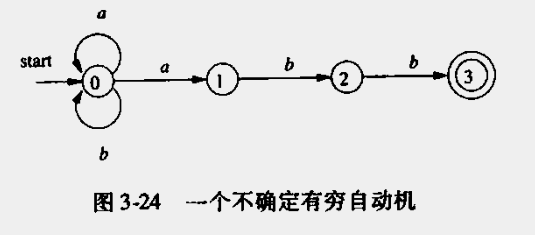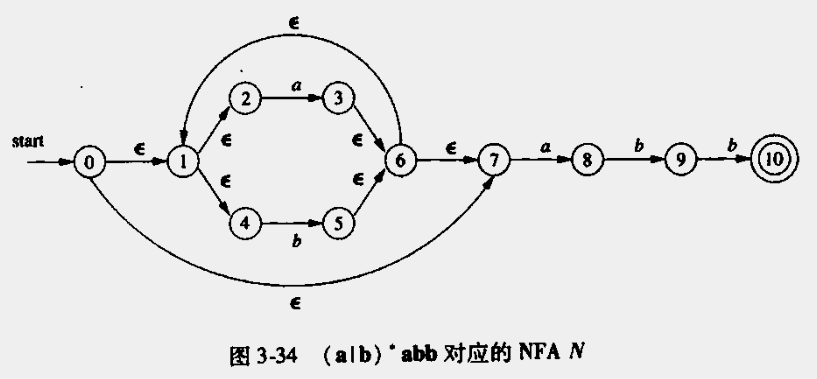## 一、引论

### 1、编译器结构

#### （1）两个阶段

• 分析（对应编译器前端）
• 输入：源程序
• 输出：中间表示形式+符号表
• 综合（对应编译器的后端）
• 输入：中间表示+符号表
• 输出：目标程序

#### （2）主要步骤

• 词法分析
• 输入：源程序字符串流
• 输出：每个词素产生一个语法单元（一个元组`<token-name, attribute-value>`）+符号表
• 语法分析
• 输入：语法单元序列+符号表
• 输出：语法树+符号表
• 语义分析
• 输入：语法树+符号表
• 职责：类型检查、自动类型转换
• 输出：语法树+符号表
• 中间代码生成
• 输入：语法树+符号表
• 输出：中间表示（三地址代码）+符号表
• 机器无关优化器（可选）
• 代码生成器
• 输入：中间表示（三地址代码）+符号表
• 输出：机器码序列
• 机器相关优化器

• 符号表贯穿始终
• 优化器可选，要保证正确前提下，提供较好的优化
• 前端部分为中间代码生成之前的部分
• 后端部分为中间代码生成之后的部分

## 二、一个简答的语法制导翻译器

### 2、语法定义

• 形式：`if(expr) stmt else stmt`
• 构造规则：`stmt → if(expr) stmt else stmt`
• `→`读作：可以有如下形式，这种规则称为产生式
• 关键字叫做：终结符号
• 变量叫做：非终结符号

#### （1）上下文无关文法定义

• 一个终结符号集合（词法单元，类似于宏替换后的最终形式）
• 一个非终结符号集合（变量，可以被替换的对象）
• 一个产生式集合（替换规则）
• 指定一个非终结符号作为开始符号

```list -> list + digit
list -> list - digit
list -> digit
digit -> 0 | 1 | 2 | 3 | 4 | 5 | 6 | 7 | 8 | 9```

```list -> list + digit | list - digit | digit
digit -> 0 | 1 | 2 | 3 | 4 | 5 | 6 | 7 | 8 | 9```
• 文法开始符号为 list
• 终结符号为 `+ - 0 1 2 3 4 5 6 7 8 9`
• 非终结符号为 `list digit`
• 空字符传定义为`ε`

#### （2）推导

```call -> id ( optparams )
optparams -> params | ε
params -> params , param | param```

#### （3）语法分析树

• 根节点为文法开始符号
• 每个叶子节点为终结符号或者`ε`
• 每个非叶子节点（内部节点）为非终结符号
• `A->XYZ`绘制成树
• A是根节点
• X是从左到右第一个树
• Y是从左到右第二个树
• Z是从左到右第三个树

#### （4）二义性

`string = string + string | string - string | 0 | 1 | 2 | 3 | 4 | 5 | 6 | 7 | 8 | 9`

#### （5）运算符的结合性

`left -> left op id | id`

`right -> id op right | id`
• id为标识符
• op为操作符

#### （6）运算符优先级

```expr -> expr + term | expr - term | term
term -> term * factor | term / factor | factor
factor -> digit | (expr)```
• expr 为表达式
• term 为单项式
• factor为因子

• 将打的概念抽象为小的概念的结合，概念的划分按照优先级最低的符号划分，层层划分直到终结因子
• 比如`+-*/`优先级最低为+-，所以：
• 一个表达式由多个单项式`+-`结合组成
• 一个单项式由多个因子`*/`结合而成
• 一个因子为一个数字或者一个`(表达式)`

### 4、语法分析

• 自顶而下（受欢迎：因为效率高）
• 自低向上（效率低，通用性高）

#### （1）自底而下的方法

• 为标号A选择一个产生式，并为该产生式中的各个符号构造出N个子节点（如何选择需要讨论：就是一种试错过程）
• 寻找分析书的左边的未被扩展的非终结符号作为A再次执行上一步，递归下去

```stmt -> expr;
|   if(expr) stmt
|   for(optexpr; optexpr;optexpr) stmt
|   other
optexpr -> ε
|   expr```

`for(;expr;expr) other`

### 5、简单的表达式翻译器

#### （2）消除左递归

`A -> Aα|Aβ|γ` 转化为等价形式

```A -> γR
R -> αR | βR | ε```

```expr -> expr + term
|   expr - term
|   term
term -> 1|2|3|4|5|6|7|8|9|0```

```expr -> term rest
rest -> + term rest
|   - term rest
|   ε
term -> 1|2|3|4|5|6|7|8|9|0```

Java版的一个实现

``````package cn.rectcircle.dragonbook.ch02;

import java.io.IOException;

/**
* 十以内自然数+-法 原始文法
*
* <pre>
* expr -> expr + term
*      |   expr - term
*      |   term
* term -> 1|2|3|4|5|6|7|8|9|0
* </pre>
*
* 消除左递归后的文法
*
* <pre>
* expr -> term rest
* rest -> + term rest
*      |  - term rest
*      |  ε
* term -> 1|2|3|4|5|6|7|8|9|0
* </pre>
*/
class Parser {

public Parser() throws IOException {
}

/**
* 非终结符号expr
*/
void expr() throws IOException {
term();
rest();
}

/**
* rest
*/
void rest() throws IOException {
// 尾递归，可以优化为循环
match('+'); term(); System.out.print('+'); rest();
match('-'); term(); System.out.print('-'); rest();
} else if(lookahead=='\n' || lookahead == -1) { // 相当于空串 ε 流式处理的终结符
return;
} else {
throw new Error("syntax error");
}
}

/**
* 终结符号数字
*/
void term() throws IOException {
} else {
throw new Error("syntax error");
}
}

/**
* 移动游标
*/
void match(int t) throws IOException {
else throw new Error("syntax error");
}
}

/**
* 解析10以内自然数加减法是否存在语法错误，并转换为后缀表达式并输出
*/
public class Postfix {
public static void main(String[] args) throws IOException {
Parser parser = new Parser();
parser.expr();
System.out.println();
}
}``````

### 6、词法分析

```expr -> expr + term
|   expr - term
|   term

term -> term * factor
|   term / factor
|   factor

factor -> (expr)
|   num
|   id```

• 一般的词法分析器都会去除空白和注释
• 预读：分析存在元素大于一个字符时
• 常量（字面量）：需要将常量比如说数字转换为一个词法单元（元组）`<num, 12>`
• 识别关键字和标识符：关键字类似`for``if`，标识符值变量名等，通常看作终结符号，比如`<id, "count">``<id, "for">`

``````package cn.rectcircle.dragonbook.ch02.lexer;

/**
* Token词法单元父类
*/
public class Token {
public final int tag;

public Token(int tag) {
this.tag = tag;
}
}``````
``````package cn.rectcircle.dragonbook.ch02.lexer;

/**
* 标签枚举
*/
public class Tag {
public final static int NUM = 256;
public final static int ID = 257;
public final static int TRUE = 258;
public final static int FALSE = 259;
}``````
``````package cn.rectcircle.dragonbook.ch02.lexer;

/**
* 数字词素
*/
public class Num extends Token{
public final int value;

public Num(int value) {
super(Tag.NUM);
this.value = value;
}
}``````
``````package cn.rectcircle.dragonbook.ch02.lexer;

/**
* 关键字or保留字词素
*/
public class Word extends Token {
public final String lexeme;

public Word(int tag, String lexeme) {
super(tag);
this.lexeme = lexeme;
}
}``````
``````package cn.rectcircle.dragonbook.ch02.lexer;

import java.io.IOException;
import java.util.HashMap;
import java.util.Map;

/**
* 四则运算词法分析器，实现的文法如下
*
* <pre>
expr -> expr + term
|   expr - term
|   term

term -> term * factor
|   term / factor
|   factor

factor -> (expr)
|   num
|   id
* </pre>
*/
public class Lexer {
public int line = 1;
private char peek = ' ';
/**
* 关键字/保留字/标识符保持单例
*/
private Map<String, Word> words = new HashMap<>();

public Lexer() {
reserve(new Word(Tag.TRUE, "true"));
reserve(new Word(Tag.FALSE, "false"));
}

/**
* 将关键字词/标识符素放入
*/
private void reserve(Word t) {
words.put(t.lexeme, t);
}

public Token scan() throws IOException {
// 处理多余空白字符
for (;; peek = (char) System.in.read()) {
if (peek == ' ' || peek == '\t') {
continue;
} else if (peek == '\n') {
line++;
} else {
break;
}
}
// 下面根据第一个有效字符的情况进行预读
// 第一个字符是数字：解析成int
if (Character.isDigit(peek)) {
int v = 0;
do {
v = 10 * v + Character.digit(peek, 10);
} while (Character.isDigit(peek));
return new Num(v);
}
// 第一个字符是字母
if (Character.isLetter(peek)) {
StringBuilder sb = new StringBuilder();
do {
sb.append(peek);
} while (Character.isLetterOrDigit(peek));
String s = sb.toString();
Word w = words.get(s);
if (w != null) {
return w;
}
w = new Word(Tag.ID, s);
reserve(w);
return w;
}
Token t = new Token(peek);
peek = ' ';
return t;
}

}``````

### 7、符号表

``````package cn.rectcircle.dragonbook.ch02.symbols;

/**
* 符号
*/
public class Symbol {

}``````
``````package cn.rectcircle.dragonbook.ch02.symbols;

import java.util.HashMap;
import java.util.Map;

/**
* 符号表（作用域），是一个树形结构。 每个符号表为一个节点，其存在一个指向父节点（上层作用域）的指针
*/
public class Env {
private Map<String, Symbol> table;
protected Env prev;

public Env(Env prev) {
this.table = new HashMap<>();
this.prev = prev;
}

public void put(String s, Symbol sym) {
table.put(s, sym);
}

public Symbol get(String s) {
for (Env e = this; e != null; e = e.prev) {
Symbol sym = e.table.get(s);
if (sym != null) {
return sym;
}
}
return null;
}

}``````

• 初始化一个top作用域符号表，作为`current`
• 每当遇到一个`{`则创建一个新的作用域，其`prev`为当前`current`，并赋值给`current`
• 每当遇到一个`}``current=current.prev`

### 8、生成中间代码

#### （1）中间代码的两种表达形式

• 树形结构（抽象语法树）
• 线性表达方式（三地址代码）
• 方便进行优化

#### （2）语法树的构造

```program -> block
block   -> '{' stmts '}'
stmts   -> stmts stmt
|  ε
stmt    -> expr;
|  if (expr) stmt
|  while (expr) stmt
|  do stmt while(expr);
|  block
expr    -> rel = expr
|  rel
|  term
term    -> term * factor
|  factor
factor  -> (expr)
|  num```
• `stmt` 为一个语句
• `expr` 为一个表达式

• Node是所有类的祖宗类，包含一个属性`n`
• Stmt是Node的子类，代表语句块
• Seq是Stmt的子类
• If等是Stmt的子类
• Eval是Stmt子类
• Expr是Node的子类，代表表达式
• Assign = 赋值
• Cond && 条件
• Rel < 比较
• Op +、- 二元运算
• Not ! 非
• Minus - 负
• Access [] 访问

• 语法检查
• 类型检查

#### （4）三地址码

`x = y op z`

```x[y] = z
x = y[z]```

```ifFalse x goto  L
ifTrue x goto  L
goto L```

`x = y`

### 9、第二章总结

• 构造一个语法直到翻译器要从源语言的文法开始。文法定义见上
• 表述一个翻译器附加属性很重要，比如变量的名字，字面量的值
• 词法分析逐一输入字符构建出词法单元（一个元组比如`<id, 'name'>`）流，翻译器使用符号表存放保留字和已经遇到的标识符
• 语法分析要解决的是从一个文法开始符号推导出终结符号串，概念上会创建一个`语法分析树`，根节点为文法开始符号，每个非叶子节点代表一个产生式，语法分析树的叶子节点从左到右组成终结符号串
• 预测分支法，采用自定而下方法可以建立高效的语法分析器。
• 语法制导翻译，通过在文法中添加规则或程序片段完成
• 语法分析结果是源代码一种中间结果，称为中间代码，有两种表现形式`抽象语法树``三地址代码`
• 符号表用于存放标识符信息，声明时放入符号表，遇到时再使用

## 三、词法分析

### 1、词法分析器的作用

• 读取源程序
• 识别次数
• 过滤注释和空白
• 生成错误消息与源程序位置关联

• 扫描阶段：删除注释和压缩空白字符
• 词法分析阶段：生成词法单元

• 简化设计，专注于一个任务，有助于设计语法
• 提高编译器的效率
• 增强编译器可移植性

• 词法单元：由一个词法单元名和一个可选属性组成
• 名称表示某种词法单元的抽象符号，比如关键字、字符序列
• 模式：描述一个词法单元的词素可能具有的形式（识别一个字符序列是某词法单元的定义）
• 词素：源程序的一个字符序列，和某个词法单元匹配，并被词法分析器识别为该词法单元的一个实例

if字符i,fif
else字符e,l,s,eelse
comparison>, < 等<=,!=
id字母开头的串pi
number任何数字常量3.124,1
literal两个”“之间除了”“之外的任意字符“string”

• 每个关键自由一个词法单元，其模式是其本身
• 表示运算符的词法单元，其模式是其本身
• 一个表示所有标识符的词法单元
• 一个或多个常量的词法单元，如数字或者字面量字符串
• 每一个标点符号有一个词法单元，比如左右括号，逗号，分号

• 比如标识符词法单元应该有一个属性，该属性是指向符号表的一个指针

### 2、输入缓冲

• 每个缓冲区的大小一般是一个磁盘块的大小
• 一般采用多缓冲
• 程序维护了两个指针
• lexemeBegin：指向当前词素的开头位置
• forword指针：一直向前扫描，直到发现一个模式为止，如果forword处理到缓冲区尾部，将文件读取接下来的文件到下一个缓冲区内，一旦与某个模式匹配，则进行词素处理。然后将lexemeBegin指向forword的下一个位置。

### 3、词法单元规约

• 字母表（Alphabet）是一个有限的符号集合，比如ascii码、Unicode码
• 串（String）是字母表中符号的一个有穷序列（其他的表述为：句子、字）
• `s` 的长度一般表示为 `|s|`
• 空串一般用 `ε` 表示
• 前缀（prefix）
• 后缀（suffix）
• 子串（substring）
• 真前缀、真后缀、真子串：排除空串的集合
• 子序列（subsequence）：从串中取出任意0个或者多个符号
• 串的运算：假设有 x = dog 、 y = house
• 连接，记做 `xy` ，则 `xy = doghouse`
• `ε` 是连接运算的单位元，任意串s，有 `sε = εs = s`
• 乘积，记做 `s^i` i为阶数
• `s^0 = ε`
• `s^i = s^(i-1) s`
• 语言（language）是给定字母表上任意一个有穷串的集合
• 语言的例子
• `∅`
• `{ε}`
• 所有语法正确的C程序的集合

• 并运算 `L∪M = {s | s ∈ L 或 s ∈ M}`
• 连接运算 `LM = { st | s ∈ L 且 t ∈ M}`
• Kleene闭包（closure）`L^* = L 连接0次或者多次得到集合`
• `L^0 = {ε}`
• 正闭包（closure）`L^+ = L 连接1次或者多次得到集合`

• `L∪D` 这个语言包含62个长度为1的串
• `LD` 包含520长度为2的串的集合且首字母为字母
• `L^4` 四个字母构成的串集合
• `L^*` 所有字母构成的串的集合，包括空串
• `L(L∪U)^*` 所有以字母开头，由数字和字母开头构成的串的集合
• `D^+` 由一个或多个数位构成的串的集合

• 归纳基础
• `ε` 是正则表达式，则 `L(ε) = { ε }`，即该语言只包含一个空串
• 如果 `a` 是 某字母表的一个符号，则 `L(a) = { a }`
• 归纳步骤
• `(r)|(s)` 是一个正则表达式，表示语言 `L(r)∪L(s)`
• `(r)(s)` 是一个正则表达式，表示语言 `L(r)L(s)`
• `(r)^*` 是一个正则表达式，表示语言 `(L(r))^*`
• `(r)` 是一个这则表达式，表示语言 `L(r)`，加括号不会影响表示的语言，只是表示优先级

• 一元运算符具有最高优先级，且左结合
• 连接操作具有次高优先级，也是左结合
• `|` 拥有最低优先级，也是左结合

• `(a) | ((b)*c)` 等价于 `ab*c`

• `r|s = s|r` 表示 `|` 满足交换律
• `r|(s|t) = (r|s)|t` 表示 `|` 满足结合律
• `r(st) = (rs)t` 表示 连接 满足结合律
• `r(s|t) = rs|rt; (s|t)r = sr|tr` 表示 `|` 满足分配率
• `εr = rε =r` 表示 `ε` 是单位元
• `r* = (r|ε)*` 表示闭包（`*`） 一定包含`ε`
• `r** = r*` 表示闭包（`*`）满足幂等性

```letter_ -> A|B|C|...|Z|a|b|...|z|_
digit   -> 0|1|2|...|9
id      -> letter_(letter_|digit)*```

`*inx` 类系统自带一个软件Lex最早使用的是一种正则表达式的扩展，本质上扩展的正则表达式都可以转化为经典正则表达式

• 一个或多个实例，使用后缀 `+` 号。表示正闭包，和 `*` 具有相同的优先级和结合性。存在如下两个代数定律
• `r* = r+|ε`
• `r+ = rr* = r*r`
• 零个或一个实例，使用后缀 `?` 号。和 `*` 具有相同的优先级和结合性。
• `r? = r|ε`
• 字符类，一个正则表达式 `a1|a2|...|an` 缩写为 `[a1a2...an]`，当序列在编码表中是连续的话可以简写为 `[a1-an]`

Lex 全部语法如下

c单个非运算字符ca
\c字符c的字面值（转义）\*
“s”串s的字面值（转义）“**”
.除换行符外任意字符a.*b
^一行的开始^abc
`\$`一行的结尾`abc\$`
`[s]`字符串s中的任意字符`[abc]`
r*0个或多个a*
r+1个或多个a+
r?0个或1个a?
r{m,n}最少m个最多n个a{1,5}
r1r2r1连接r2ab
`r1r2`r1并r2
®和r相通(a)
r1/r2后面跟有r2时的r1abc/123

```letter_ -> [A-Za-z_]
digit   -> [0-9]
id      -> letter_(letter_|digit)*```

### 4、词法单元的识别

• 状态转换图

KMP算法：

• 计算trie失效函数：f(s)
• s为失效的地方（从1开始）
• f(s)的含义：满足 `b[0:len] == b[s-len:s]``f(s) = max(len)`
• 使用模式串进行匹配，如果失效后，模式串向右移动`s-f(s)`字符

`b="ababaa"`的失效函数

s123456
f(s)001231
b[s-1]ababaa

``````b = "ababaa";
t = 0; // 前缀的长度（下一个字符的下标）
f = 0;
for(int s=1; s<n; s++){ // 此s表示b的下标（从0开始）
while(t>0 && b[s] != b[t]) t = f[t]; //找到一个最长前缀==后缀，直到长度为0。
if(b[s] == b[t]){ t=t+1; f[s+1] = t;} // 如果t==0 此句不执行
else f[s+1] = 0;
}``````

``````a = "fabababaa";
s = 0;
for(int i=0; 0<m; i++){
while(s>0 && a[i] != b[s]) s = f[s];  //发生失配，一直进行回退，直到s==0
if(a[i] == b[s]) s = s + 1; // 如果回退到了s==0，此不执行，直接跳到i++
if(s == n) return i;
}
return -1;``````

KMP算法扩展：使用多模式串，例如下待匹配的模式串

• `he`
• `she`
• `his`
• `hers`• 给每个节点编号，用于失效函数
• 两个圆圈表示终结点，当匹配到该字符则直接返回（如果在中间需要考虑是否贪婪）

s123456789
f(s)000120303

### 6、有穷自动机

• 对输入只能简单回答是或否
• 分为两类
• 不确定有穷自动机（NFA）：一个符号可以标记离开该状态的多条边
• 确定的有穷自动机（DFA）：一个符号只能标记离开该状态的一条边

#### （1）不确定的有穷自动机

• 一个有穷的状态集合`S`
• 一个输入符号集合`∑`，即输入字母表，假设空串`ε`不是`∑`的元素
• 一个状态转换函数，它为`∑∪{ε}`中每个符号都给出了相应的后记状态的集合
• S中的一个状态`s0`指定为`开始状态`
• S的一个子集F被指定为`终止状态`0{0,1}{0}
1{2}
2{3}
3

#### （2）确定的有穷自动机

• 没有ε之上的转换动作
• 每个状态s和输入符号a，只有一条边离开s### 7、从正则表达式到自动机

• 使用DFA进行匹配
• 使用NFA进行匹配

#### （1）从NFA到DFA的转换

• 输入：NFA N
• 输出：DFA D

• `N`：表示NFA图
• `D`：表示DFA图
• `Dstates`：D的状态集合，每个元素是T，和是否被标记
• `Dtran`转换表：是D的表现形式。每一行是包含如下信息
• `D`的当前状态`T`和经过输入符号，到达的状态U的映射，记做 `Dtran[T,a]`
• `D`的当前状态和`N`的状态集合的对应关系
• `s`：表示N的一个状态
• `s0`：表示开始状态
• `T,U,A,B,C`：表示N某些状态的集合，同时表示D的一个状态
• `F`: 终止状态集合
• `a`：表示边标号输入符号集合中的一个元素
• `0,1,2,3`：表示N的状态编号
• 核心函数
• `ε-closure(s)`：表示从状态s出发，只经过`ε`边可以到达的所有节点
• `ε-closure(T)`：表示从T中的任意状态s出发，只经过`ε`边可以到达的所有状态的集合
• `move(T,a)`：表示从T中的任意状态s出发，只经过`a`可以到达的所有状态的集合
• 本质上是一个函数：`ε-closure(s) = ε-closure({s}) = move({s}, ε)`：实现上就是图的遍历

```Dstates = {ε-closure(s0)} // 不添加标记
while(Dstates中存在未标记的元素){
T = Dstates中未标记的元素
for(针对每个输入符号){
U = ε-closure(move(T,a))
if(U not in Dstates)
将U添加到Dstates中并不添加标记
DTran[T, a] =U
}
}```

NFA表示1• 步骤1：`A = ε-closure(0) = {0, 1, 2, 4, 7}` （一定包括0，0为起点可以通过ε到达自身），将`A`添加到`Dstates`
• 步骤2：
• 从Dstates中取出A，将A进行标记
• `Dtran[A, a] = ε-closure(move(A, a)) = ε-closure({3, 8}) = {1, 2, 3, 4, 6, 7, 8} = B` (因为不在`Dstates`中)，并添加到`Dstates`
• `Dtran[A, b] = ε-closure(move(A, b)) = ε-closure({5}) = {1, 2, 4, 6, 7} = C` (因为不在`Dstates`中)，并添加到`Dstates`
• 步骤…

NFA状态DFA状态ab
{0, 1, 2, 4, 7}ABC
{1, 2, 3, 4, 6, 7, 8}BBD
{1, 2, 4, 6, 7}CBC
{1, 2, 4, 5, 6, 7, 9}DBE
{1, 2, 4, 5, 6, 7, 10}EBC

NFA表示2NFA状态DFA状态ab
{0}ABA
{0, 1}BBC
{0, 2}CBD
{0, 3}DBA

#### （2）NFA的模拟

```S = ε-closure(s0)
c = nextC()
while(c != eof){
S = c-closure(move(S,c))]
c = nextChar()
}
if( |S∩F| != 0) return true
else return false```

#### （3）NFA的效率

`O(k(m+n))`

• k ：带匹配字符串的长度
• n：状态数
• m：边数